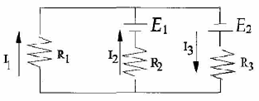# Problem: The following equation is true for the right loop of the circuit. a) E2 - I3R3 - I2R2 - E1 = 0 b) -E2 - I3R3 - I2R2 - E1 = 0 c) E2 - I3R3 - I2R2 + E1 = 0 d) E2 + I3R3 + I2R2 - E1 = 0 e) None of the above

###### FREE Expert Solution
97% (386 ratings)
###### Problem Details

The following equation is true for the right loop of the circuit.

a) E2 - I3R3 - I2R2 - E1 = 0

b) -E2 - I3R3 - I2R2 - E1 = 0

c) E2 - I3R3 - I2R2 + E1 = 0

d) E2 + I3R3 + I2R2 - E1 = 0

e) None of the aboveFrequently Asked Questions

What scientific concept do you need to know in order to solve this problem?

Our tutors have indicated that to solve this problem you will need to apply the Kirchhoff's Loop Rule concept. You can view video lessons to learn Kirchhoff's Loop Rule. Or if you need more Kirchhoff's Loop Rule practice, you can also practice Kirchhoff's Loop Rule practice problems.